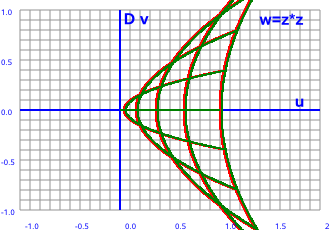# Maths - Functions Of Double Numbers

On this page we discuss how to extend the scalar functions (that we discussed on this page) to the situation where the domain and/or codomain are double numbers, we also discuss functions like normalise.

## Conjugate

As with complex numbers when we multiply a double number by its conjugate we get a real number, in other words the imaginary part cancels out. However the value is different:

for complex numbers: (a + i b)(a - i b) = a² + b²

for double numbers: (a + D b)(a - D b) = a² - b²

This is the Minkowski norm.

## Inverse Function

To calculate the inverse value (1/z) we multiply the top and bottom by the conjugate which makes the denominator a real number.

z plane   w plane-->

w=1/zhow this plot was produced.

Let the components of the input and output planes be:

z = x + D y and w = u + D v

In this case w = 1/z

so:

w = 1/(x + D y)

As usual, we evaluate the inverse by multiplying top and bottom by the conjugate:

w = (x - D y)/(x + D y)(x - D y)

w = (x - D y)/(x² - y²)

so the u and v components are:

u = x /(x²-y²)
v = -y /(x²-y²)

## Evaluating Double Functions

We can evaluate the value of many double functions by using infinite series in the same way that we can for scalar functions. We just use the same series that we would use for scalar functions but plug in double values instead of real values. So if a series exists for real values we can evaluate the double number function.

## Exponential Function

This is discussed when we discuss polar form and Euler's equation on this page.

z plane   w plane-->

w=ezhow this plot was produced.

Let the components of the input and output planes be:

z = x + D y and w = u + D v

In this case w = ez

so:

w = e(x + D y)

by the rules of exponents:

w = exeD y

How do we evaluate this? We can use the hyperbolic version of Euler's equation which gives:

w = ex(cosh(y) + D sinh(y))

More about hyperbolic trig functions here.

so the u and v components are:

u = excosh(y)
v = exsinh(y)

## Log Function

The natural log, that is the log to the base e, is the inverse of the exponetial function. So if,

w = ln(z)

then taking the exponent of each side gives,

ew = z

so we have

x = eucosh(v)
y = eusinh(v)

which gives:

u = ln(√(x² - y²))
v = atanh(y/x)

notes: atanh expects its argument to be: -1 > arg > 1 in this case:

-1 >y/x> 1

or multiplying by x to give:

-x >y > x

sqrt expects its argument to be: 0 < arg in this case:

0 < x² - y²

which gives:

y² < x² so,

|y| < |x| which means that again:

-x >y > x

## Powers of double numbers

z plane   w plane-->

w=z²how this plot was produced.

Pure real values always square to a positive value and pure imaginary values always square to a negative value. However real and imaginary parts together cover the whole plane.

Let the components of the input and output planes be:

z = x + D y and w = u + D v

lets take the example of the square function w = z²

so:

w = (x + D y)²

multiplying out gives:

w = x² + y² + D 2 x y

so the u and v components are:

u = x² + y²
v = 2 x y

## Norm

This is the distance (r) of a + D b from the origin.

It is written as:

r = | a + D b |

by Pythagoras:

r = | a + D b | = math.sqrt(a*a + b*b)

Check that:

|a + D b|*|c + D d| = |a*c - b*d + D (a*d + b*c)|

## Further Study

I think its interesting to compare these graphs for complex numbers to the equivalent graphs for complex numbers and dual numbers.